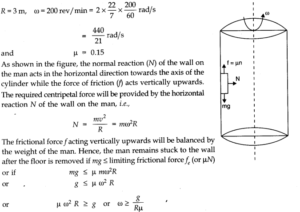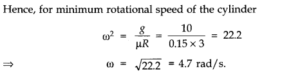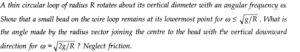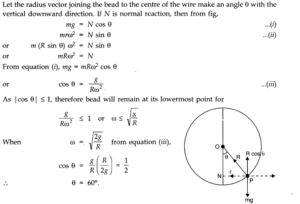# Chapter 5 – Laws of motion Questions and Answers: NCERT Solutions for Class 11 Physics

Class 11 Physics NCERT book solutions for Chapter 5 - Laws of motion Questions and Answers.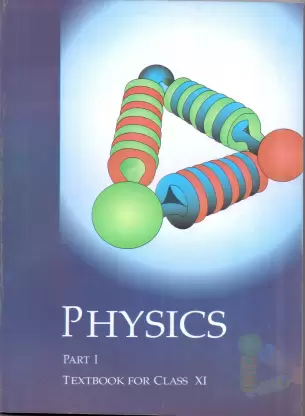## Question 5. 5. A constant retarding force of 50 N is applied to a body of mass 20 kg moving initially with a speed of 15ms-3. How long does the body take to stop? Answer:Here m = 20 kg, F = – 50 N (retardation force) As F = ma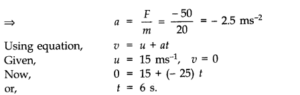## Question 5. 6. A constant force acting on a body of mass 3.0 kg changes its speed from 2.0 ms-1to 3.5 ms-1in 25 s. The direction of the motion of the body remains unchanged. What is the magnitude and direction of the force?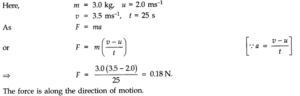## Question 5. 7. A body of mass 5 kg is acted upon by two perpendicular forces 8 N and 6 N. Give the magnitude and direction of the acceleration of the body.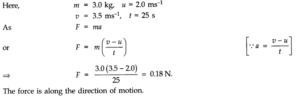## Question 5. 8. The driver of a three-wheeler moving with a speed of 36 km/h sees a child standing in the middle of the road and brings his vehicle to rest in 4.0 s just in time to save the child. What is the average retarding force on the vehicle? The mass of the three-wheeler is 400 kg and the mass of the driver is 65 kg.

### Answer:Here mass of three-wheeler mi = 400 kg, mass of driver = m2= 65 kg, initial speed of auto, u = 36 km/h = 36 x — m/s 10 ms-1, final speed, v – 0 and t = 4s.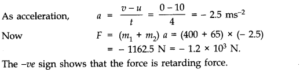## Question 5. 10. A body of mass 0.40 kg moving initially with a constant speed of 10 ms-1to the north is subject to a constant force of 8.0 N directed towards the south for 30 s. Take the instant the force is applied to be t = 0, the position of the body at that time to be x = 0, and predict its position at t = -5 s, 25 s, 100 s.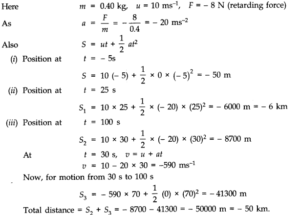## Question 5. 11. A truck starts from rest and accelerates uniformly at 2.0 ms-2. At t = 10 s, a stone is dropped by a person standing on the top of the truck (6 m high from the ground). What are the (a) velocity, and (b) acceleration of the stone at t = 11s? (Neglect air resistance.)

### Answer:u = 0, a = 2 ms-2, t 10 s Using equation, v = u + at, we get v = 0 + 2 x 10 = 20 ms-1 (a) Let us first consider horizontal motion. The only force acting on the stone is force of gravity which acts vertically downwards. Its horizontal component is zero. Moreover, air resistance is to be neglected. So, horizontal motion is uniform motion. .-. vx = v = 20 ms-1 Let us now consider vertical motion which is controlled by force of gravity. u=0, a = g = 10 ms-2, t = (11 — 10) s = 1 s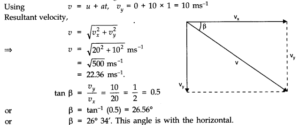## Question 5. 12. A bob of mass 0.1 kg hung from the ceiling of a room by a string 2 m long is set into oscillation. The speed of the bob at its mean position is 1 ms-1. What is the trajectory of the bob if the stringis cut when the bob is (a) at one of its extreme positions, (b) at oits mean position ?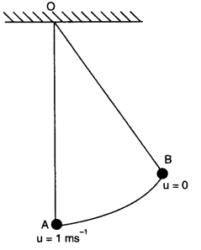## Question 5. 14. Figure shows the position-time graph of a particle of mass 4 kg. What is the (a) force on the particle for t < 0, t > 4 s, 0 < t < 4 s? (b) impulse at t = 0 and t = 4 s? (Consider one-dimensional motion only).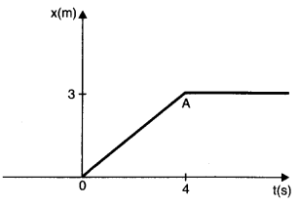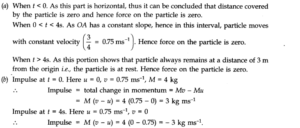## Question 5. 15. Two bodies of masses 10 kg and 20 kg respectively kept on a smooth, horizontal surface are tied to the ends of a tight string. A horizontal force F = 600 N is applied to (i) A, (ii) B along the direction of string. What is the tension in the string in each case?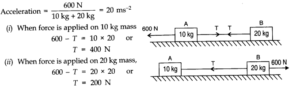## Question 5. 16. Two masses 8 kg and 12 kg are connected at the two ends of a light in extensible string that goes over a friction less pulley. Find the acceleration of the masses, and the tension in the string when the masses are released.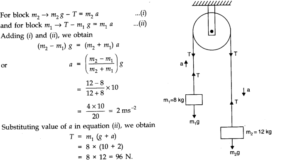## Question 5. 17. A nucleus is at rest in the laboratory frame of reference. Show that if it disintegrates into twosmaller nuclei the products must move in opposite directions.

### Answer:Let m1, m2 be the masses of products and v1,v2be their respective velocities. Therefore, total linear momentum after disintegration = m1v1+m2v2. Before disintegration, the nucleus is at rest. Therefore, its linear momentum before disintegration is zero. According to the principle of conservation of linear momentum,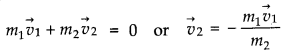## Question 5. 19. A shell of mass 0.020 kg is fired by a gun of mass 100 kg. If the muzzle speed of the shell is 80 ms-1what is the recoil speed of the gun?

### Answer:m = 0.02 kg, M = 100 kg, v = 80 ms-1, V = ?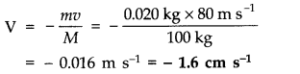## Question 5. 20. A batsman deflects a ball by an angle of 45° without changing its initial speed which is equal to 54 km/h. What is the impulse imparted to the ball? (Mass of the ball is 0.15 kg.)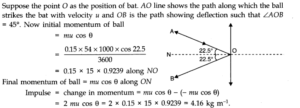## Question 5. 21. A stone of mass 0.25 kg tied to the end of a string is whirled round in a circle of radius 1.5 m with a speed of 40 rev./min in a horizontal plane. What is the tension in the string? What is the maximum speed with which the stone can be whirled around if the string can withstand a maximum tension of 200 N?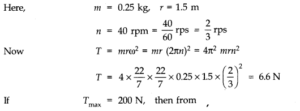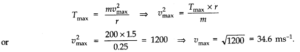## Question 5. 24. Figure shaws the position-time graph of a particle of mass 0.04 kg. Suggest a suitable physical context for this motion. What is the time between two consecutive impulses received by the particle ? What is the magnitude of each impulse?

### Answer:This graph can be of a ball rebounding between two walls situated at position 0 cm and 2 cm. The ball is rebounding from one wall to another, time and again every 2 s with uniform velocity.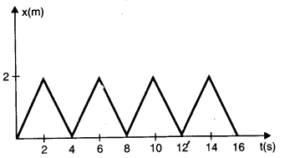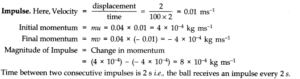## Question 5. 25. Figure shows a man standing stationary with respect to a horizontal conveyor belt that isaccelerating with 1 ms-2. What is the net force on the man ? If the coefficient of static friction , between the man’s shoes and the belt is 0.2, up to what acceleration of the belt can the man continue to be stationary relative to the belt? (Mass of the man = 65 kg.)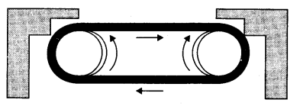### Answer:Here acceleration of conveyor belt a = 1 ms-2, μs= 0.2 and mass of man m = 65 kg. t As the man is in an accelerating frame, he experiences a pseudo force Fs= ma as shown in fig. (a). Hence to maintain his equilibrium, he exerts a force F = – Fs= ma = 65 x 1 = 65 N in forward direction i.e., direction of motion of belt. .’. Net force acting on man = 65 N (forward) As shown in fig. (b), the man can continue to be stationary with respect to belt, if force of friction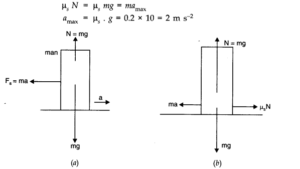## Question 5. 26. A stone of mass m tied to the end of a string is revolving in a vertical circle of radius R. The net force at the lowest and highest points of the circle directed vertically downwards are: (choose the correct alternative).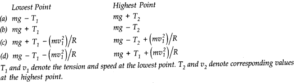## Question 5. 30. An aircraft executes a horizontal loop at a speed of 720 km/h with its wings banked at 15°. What is the radius of the loop?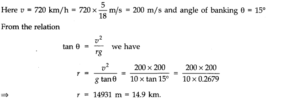## Question 5. 31. A train runs along an un banked circular track of radius 30 m at a speed of 54 km/h. The mass of the train is106kg. What provides the centripetal force required for this purpose the engine or the rails? What is the angle of banking required to prevent wearing out of the rail?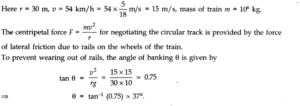## Question 5. 32. A block of mass 25 kg is raised by a 50 kg man in two different ways as shown in Fig. What is the action on the floor by the man in the two cases? If the floor yields to a normal force of 700 N, which mode should the man adopt to lift the block without the floor yielding?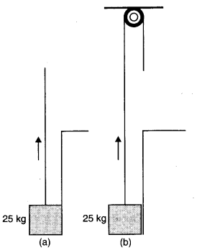## Question 5. 33. A monkey of mass 40 kg climbs on a rope (Fig.) which can stand a maximum tension of 600 N. In which of the following cases will the rope break: the monkey (a) climbs up with an acceleration of 6 ms-2 (b) climbs down with an acceleration of 4 ms-2 (c) climbs up with a uniform speed of 5 ms-1 (d) falls down the rope nearly freely under gravity? (Ignore the mass of the rope).

### Answer:(a) When the monkey climbs up with an acceleration a, then T – mg = ma where T represents the tension (figure a).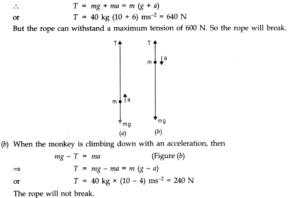## Question 5. 34. Two bodies A and B of masses 5 kg and 10 kg in contact with each other rest on a table against a rigid wall (Fig.). The coefficient of friction between the bodies and the table is 0.15. A force of 200 N is applied horizontally to A. What are (a) the reaction of the partition (b) the action-reaction forces between A and B? What happens when the wall is removed? Does the answer to (b) change, when the bodies are in motion? Ignore the difference between μs and μk.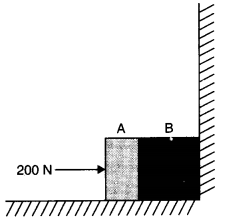### Answer:(i) When the wall exists and blocks A and B are pushing the wall, there can’t be any motion i.e., blocks are at rest. Hence, (a) reaction of the partition = – (force applied on A) = 200 N towards left. (b) action-reaction forces between A and B are 200 N each. A presses B towards right with an action force 200 N and B exerts a reaction force on A towards left having magnitude 200 N. (ii) When the wall is removed, motion can take place such that net pushing force provides the acceleration to the block system. Hence, taking kinetic friction into account, we have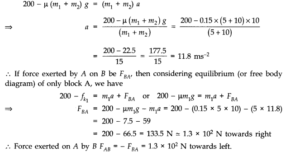## Question 5. 36. The rear side of a truck is open and a box of 40 kg mass is placed 5 m away from the open end as shown in Fig. The coefficient of friction between the box and the surface below it is 0.15. On a straight road, the truck starts from rest and accelerates with 2 ms-2. At what distance from the starting point does the box fall off the truck? (Ignore the size of the box).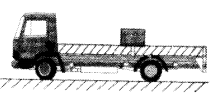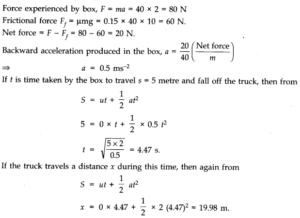## Question 5. 37. A disc revolves with a speed of 33 1/3 rpm and has a radius of 15 cm. Two coins are placed at 4 cm and 14 cm away from the centre of the record. If the coefficient of friction between the coins and record is 0.15, which of the coins will revolve with the record?

### Answer:If the coin is to revolve with the record, then the force of friction must be enough to provide the necessary centripetal force.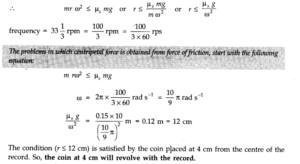## Question 5. 38. You may have seen in a circus a motorcyclist driving in vertical loops inside a ‘death well’ (a hollow spherical chamber with holes, so the spectators can watch from outside). Explain clearly why the motorcyclist does not drop down when he is at the uppermost point, with no support from below. What is the minimum speed required at the uppermost position to perform a vertical loop if the radius of the chamber is 25 m?

### Answer:When the motorcyclist is at the highest point of the death-well, the normal reaction R on the motorcyclist by the ceiling of the chamber acts downwards. His weight mg also acts downwards. These two forces are balanced by the outward centrifugal force acting on him.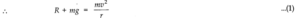### Here v is the speed of the motorcyclist and m is the mass of the motorcyclist (including the mass of the motor cycle). Because of the balancing of the forces, the motorcyclist does not fall down. The minimum speed required to perform a vertical loop is given by equation (1) when R = 0.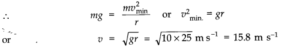## Question 5. 39. A 70 kg man stands in contact against the inner wall of a hollow cylindrical drum of radius 3 m rotating about its vertical axis with 200 rev/min. The coefficient of friction between the wall and his clothing is 0.15. What is the minimum rotational speed of the cylinder to enable the man to remain stuck to the wall (without falling) when the floor is suddenly removed?